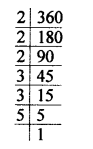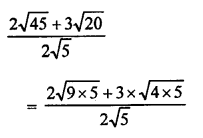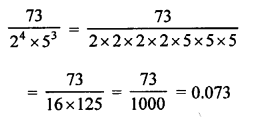## RS Aggarwal Class 10 Solutions Chapter 1 Real Numbers Ex 1E

These Solutions are part of RS Aggarwal Solutions Class 10. Here we have given RS Aggarwal Solutions Class 10 Chapter 1 Real Numbers Ex 1E.

Other Exercises

Question 1.
Solution:
For any two given positive integers a and b there exist unique whole numbers q and r such that
a = bq + r, where 0 ≤ r < b.
Here, we call ‘a’ as dividend, b as divisor, q is quotient and r as remainder.
Dividend = (Divisor x Quotient) + Remainder

Question 2.
Solution:
Every composite number can be uniquely expressed as a product of two primes, except for the order in which these prime factors occurs.

Question 3.
Solution:
360 = 2 x 2 x 2 x 3 x 3 x 5 = 23 x 3² x 5Question 4.
Solution:
We know that HCF of two primes is
HCF (a, b) = 1

Question 5.
Solution:
a and b are two prime numbers then their
LCM = Product of these two numbers
LCM(a, b) = a x b = ab.

Question 6.
Solution:
We know that product of two numbers is equal to their HCF x LCM
LCM = $$\frac { Product of two numbers }{ HCF }$$
= $$\frac { 1050 }{ 25 }$$ = 42
LCM of two numbers = 42

Question 7.
Solution:
A composite number is a number which is not a prime. In other words, a composite number has more than two factors.

Question 8.
Solution:
a and b are two primes, then their
HCF will be 1
HCF of a and b = 1

Question 9.
Solution:
$$\frac { a }{ b }$$ is a rational number and it has terminating decimal
b will in the form 2m x 5n where m and n are some non-negative integers.

Question 10.
Solution:Question 11.
Solution:Question 12.
Solution:
2n x 5n = (2 x 5)n = (10)n
Which always ends in a zero
There is no value of n for which (2n x 5n) ends in 5

Question 13.
Solution:
We know that HCF is always a factor is its LCM
But 25 is not a factor of 520
It is not possible to have two numbers having HCF = 25 and LCM = 520

Question 14.
Solution:
Let two irrational number be (5 + √3) and (5 – √3).
Now their sum = (5 + √3) + (5 – √3) = 5 + √3 + 5 – √3 = 10
Which is a rational number.

Question 15.
Solution:
Let the two irrational number be (3 + √2) and (3 – √2)
Now, their product = (3 + √2) (3 – √2)
= (3)² – (√2)² {(a + b) (a – b) = a² – b²}
= 9 – 2 = 7
Which is a rational number.

Question 16.
Solution:
a and b are relative primes
their HCF = 1Question 17.
Solution:
LCM of two numbers = 1200
and HCF = 500
But we know that HCF of two numbers divides their LCM.
But 500 does not divide 1200 exactly
Hence, 500 is not their HCF whose LCM is 1200.

Question 18.
Solution:
Let x = 0.4 = 0.444
Then 10x = 4.444….
Subtracting, we get
9x = 4 => x = $$\frac { 4 }{ 9 }$$
$$\bar { 0.4 }$$ = $$\frac { 1 }{ 2 }$$ which is in the simplest form.

Question 19.
Solution:
$$\bar { 0.23 }$$
Let x = $$\bar { 0.23 }$$ = 0.232323…….
and 100x = 23.232323……
Subtracting, we get
99x = 23 => x = $$\frac { 23 }{ 99 }$$
$$\bar { 0.23 }$$ = $$\frac { 23 }{ 99 }$$ which is in the simplest form.

Question 20.
Solution:
0.15015001500015
It is non-terminating non-repeating decimal.
It is an irrational number.

Question 21.
Solution:
$$\frac { \surd 2 }{ 3 }$$ = $$\frac { 1 }{ 3 }$$ √2
Let $$\frac { 1 }{ 3 }$$ √2 is a rational number
Product of two rational numbers is a rational
$$\frac { 1 }{ 3 }$$ is rational and √2 is rational contradicts
$$\frac { \surd 2 }{ 3 }$$ or $$\frac { 1 }{ 3 }$$ √2 is irrational.

Question 22.
Solution:
√3 and 2.
√3 = 1.732 and 2.000
A rational number between 1.732 and 2.000 can be 1.8 or 1.9
Hence, 1.8 or 1.9 is a required rational.

Question 23.
Solution:
$$\bar { 3.1416 }$$
It is non-terminating repeating decimal.
It is a rational number.

Hope given RS Aggarwal Solutions Class 10 Chapter 1 Real Numbers Ex 1E are helpful to complete your math homework.

If you have any doubts, please comment below. Learn Insta try to provide online math tutoring for you.